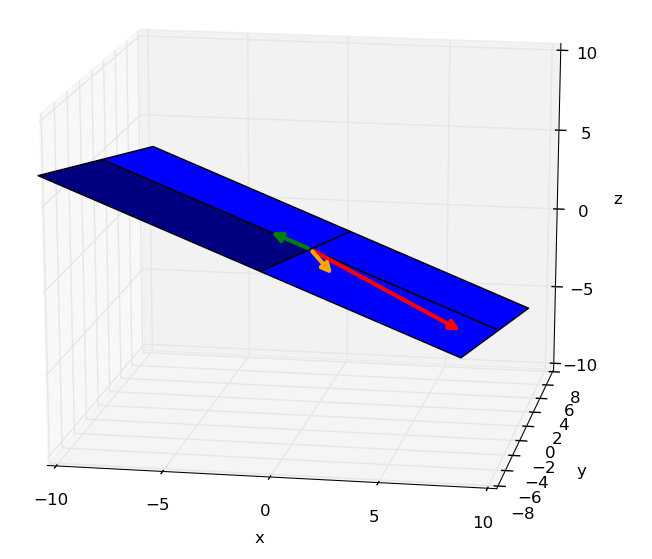## 1. Linear combination

If a vector can be expressed as a linear combination of other vectors, they are said to be linearly dependent.

#### Example IFigure 6.1 : vectors linearly dependent

We’d like to know if one vector in Figure 6.1 depends upon the others, as the following :

We have thus :

Let’s factorize the right hand-side of $(2)$ :

Let’s proceed with the matrix elimination as we have seen in the dedicated chapter :

From the matrix in echelon form, we get :

$-4\lambda_3 = 12 \iff$ $\lambda_3 = -3$

Let’s replace $\lambda_3$ in the first row :

$2\lambda_2 + -2 \cdot \underbrace{-3}_{\lambda_3} = 8 \iff$ $\lambda_2 = 1$

The solution is :

Therefore any vector $\vec{v_i} \in \{\vec{v_1}, \vec{v_2}, \vec{v_3}\}$ can be expressed as the linear combination of the others.

## 2. Generalization

$(1)$ is obviously equivalent to $(4)$ :

More generally, we have :

If the unique solution to $(5)$ is $\lambda{_1}=\lambda{_2}=\lambda{_3}=0$ then none of the vectors can be expressed as the linear combination of the other. Otherwise the vectors are linearly dependent.

## Recapitulation

If a vector can be expressed as the linear combination of other vectors, they are said to be linearly dependent. As for example :
$\left( \begin{smallmatrix} 2 \\ -1 \\ -1 \end{smallmatrix} \right) = {\small -1} \left( \begin{smallmatrix} 1 \\ -1 \\ 0 \end{smallmatrix} \right) + {\small -1} \left( \begin{smallmatrix} -3 \\ 2 \\ 1 \end{smallmatrix} \right)$.

To know if one vector $\vec{v_i} \in \{\vec{v_1}, \vec{v_2}, \ldots{} ,\vec{v_n} \}$ can be expressed as the linear combination of the others, the following equation has to be solved :

If that equation has as a unique solution all $\lambda{_i}=0$, it means that none of the vecteors $\vec{v_i}$ depends upon the others. In that case, the vectors are said to be linearly independent.